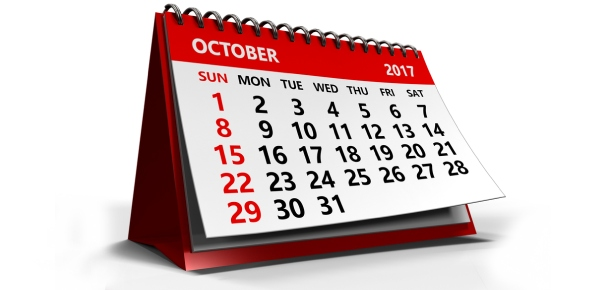# Practice October 21

18 Questions | Total Attempts: 164SettingsThis quiz is quick math. It is 6's.

Related Topics
• 1.
6*8=
• A.

48

• B.

12

• C.

68

• 2.
6*2=
• A.

8

• B.

62

• C.

12

• 3.
6*6=
• A.

66

• B.

36

• C.

12

• 4.
6*9=
• A.

37

• B.

26

• C.

54

• 5.
6*7=
• A.

47

• B.

42

• C.

48

• 6.
6*5=
• A.

30

• B.

35

• C.

25

• 7.
6*3=
• A.

24

• B.

81

• C.

18

• 8.
6*1=
• A.

12

• B.

6

• C.

78

• 9.
6*4=
• A.

24

• B.

42

• C.

12

• 10.
6*11=
• A.

65

• B.

67

• C.

66

• 11.
6*10=
• A.

60

• B.

4

• C.

71

• 12.
6*12=
• A.

64

• B.

2

• C.

72

• 13.
6*8=
• A.

43

• B.

48

• C.

81

• 14.
6*2=
• A.

42

• B.

21

• C.

12

• 15.
6*9=
• A.

45

• B.

54

• C.

66

• 16.
6*7=
• A.

42

• B.

69

• C.

65

• 17.
6*11=
• A.

55

• B.

77

• C.

66

• 18.
6*12=
• A.

72

• B.

27

• C.

34